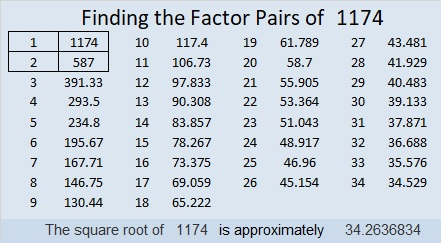# 1174 and Level 1

I’ve given you just nine clues in this puzzle, but that’s enough to find all the factors AND complete the entire table. I’m serious. I really have given you sufficient information to find the one and only solution to this puzzle!Print the puzzles or type the solution in this excel file: 10-factors-1174-1186

Now I’ll share some information about the number 1174:

• 1174 is a composite number.
• Prime factorization: 1174 = 2 × 587
• The exponents in the prime factorization are 1 and 1. Adding one to each and multiplying we get (1 + 1)(1 + 1) = 2 × 2 = 4. Therefore 1174 has exactly 4 factors.
• Factors of 1174: 1, 2, 587, 1174
• Factor pairs: 1174 = 1 × 1174 or 2 × 587
• 1174 has no square factors that allow its square root to be simplified. √1174 ≈ 34.263681174 is also the sum of the sixteen prime numbers from 41 to 107.

This site uses Akismet to reduce spam. Learn how your comment data is processed.